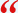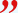# Energy Distribution of Radiation Emitted by a Black-Body- Independent of ProbabilityAn article is published in Indian journal of Science and Technology, Vol. 9, Issue 45, December 2016, proposing that energy distribution of radiation emitted by a black-body has an alternative explanation independent of probability.• (1888PressRelease) December 24, 2016 - Panchkula, Haryana, India - Towards the end of the nineteenth century, with the advent of incandescent electric bulbs, a need was felt to investigate the emission of light from electrically heated filament of bulb so as to give the best result with minimum input of electrical energy. This requirement led scientists to experiment with radiation emitted from the black-body as this is identical to radiation emitted by a luminous bulb.

Data was compiled for radiation emitted at various frequencies and temperatures of the black-body. From that data, radiation curves for energy emitted over a wide range of wavelength at different absolute temperatures were drawn and analysis of the data helped German physicist Max Planck, to find a formula for the radiation curves.

However, there was no satisfactory explanation for the formula until Max Planck explained it on the basis of his idea of quantization of energy. In his own words, the explanation for this formula was an 'Act of desperation' because he had to use probability and quantization of energy for deriving the formula, whereas he was a staunch critic of both. He hoped that someday he will give the explanation without probability and quantization. But as the time passed, the idea of quantization became so useful to physics that it became a branch of science known as Quantum Physics. Since probability was put into use in physics at the same time as quantization, it grew with Quantum Physics and led to many weird results.

Author, Devinder Dhiman, writes in the article that there is no need to consider the oscillators and modes of waves of electromagnetic radiations inside the black-body to derive the formula of energy distribution of radiation emitted by the black-body. He compares the energy released by radiations emitted from the cavity of a black-body with the energy released from an incandescent electric bulb. Electrical energy added to the bulb is then envisaged to transform into energy-sphere that breaks-up into small packets of energy akin to radioactive decay of matter, and generates electromagnetic radiations causing brightness. Derivation of the formula, in this method, does not involve use of probability.

The article has importance in the fact that since 1900, the explanation of the formula based on probability has been prevalent in physics; it is for the first time that an explanation has been given without the use of probability.

Contact:
Devinder Dhiman
Authordevinderdhiman.com
House no. 254, Sector 21
Panchkula, Haryana
+919814910285
dhimandk ( @ ) rediffmail dot com
http://www.authordevinderdhiman.com

###
space
space
Contact Information